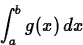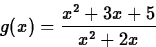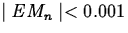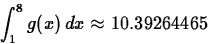# Exercises

1.
Consider the function f(x) = 4-x on the interval [0,4]. Verify that the middlesum command gives the exact value for the area independent of the number of subintervals you specify. Can you explain this? Note that you can use the formula for the area of a triangle to compute that the area is 8. (Hint - look at the plot produced by the middlebox command.)
2.
Consider the general linear function g(x) = cx+d, where c and d are constants. What does the error bound formula say about the accuracy of the midpoint rule for approximatingDoes this make sense, based on your result for the first exercise? Explain.

3.
Consider the function f(x) = x4-5x3+12x2-x+1 on the interval [0,5]. Use the command leftsum to approximate the definite integralto two decimal places. Looking at the graph of f, can you explain why value given by the leftsum command is always less than the value of the integral? If you used the rightsum command, to approximate this same integral, do you think your approximations would be smaller than the value of the integral, larger than the integral, or could it be larger or smaller depending on the number of subintervals you use? Explain your answer.

4.
Consider the functionon the interval [1,8].
(a)
Use the error bound formula to find the smallest value of n that guarantees that Mn approximates the area to within 0.001. That is, find the smallest value of n that guarantees that.
(b)
The value of n given by the error bound is usually conservative. That is, in practice the desired accuracy can be achieved with a smaller value of n. Given thatfind the smallest value of n such that.Next: About this document ... Up: Labs and Projects for Previous: Area Approximations

Jane E Bouchard
3/24/2000